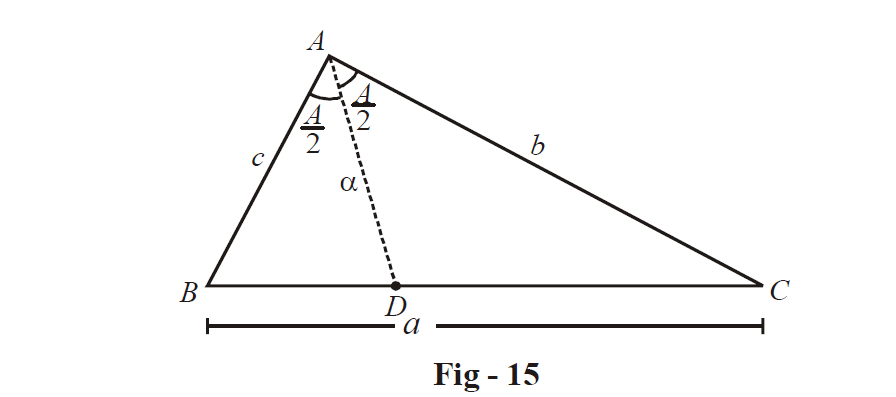# Examples on Sine Rule Set 3

Go back to  'Trigonometry'

Example – 40

Find the ratio a : b : c in a  $$\Delta ABC$$  : if

$\cos A\cos B + \sin A\sin B\sin C = 1$

Solution: Note that

\begin{align}&\sin C = \frac{{1 - \cos A\cos B}}{{\sin A\sin B}} \leqslant 1 \\ \Rightarrow\qquad &\sin A\sin B + \cos A\cos B = \cos (A - B) \geqslant 1\\ \end{align}

This necessarily implies that

\begin{align}&\cos (A - B) = 1\\ \Rightarrow\qquad &\angle A = \angle B \\ \end{align}

\begin{align}&\Rightarrow \quad \sin C = \frac{{1 - {{\cos }^2}A}}{{{{\sin }^2}A}} = 1 \quad \Rightarrow\quad C = \frac{\pi }{2} \\\,\,\,\,\,\,\,\,\,\,\,\, &\qquad\qquad\qquad\Rightarrow A = B = \frac{\pi }{4} \\ \end{align}

Since \begin{align}\frac{a}{{\sin A}} = \frac{b}{{\sin B}} = \frac{c}{{\sin C}}\end{align}  from the sine rule, we have

\begin{align}&\frac{a}{{1/\sqrt 2 }} = \frac{b}{{1/\sqrt 2 }} = \frac{c}{1} \\ \Rightarrow\qquad &a:b:c = 1:1:\sqrt 2 \\ \end{align}

Example - 41

Let  $$\alpha ,\;\beta ,\;\gamma$$   be the lengths of the internal angle bisectors of angles A, B, C respectively in a  $$\Delta ABC$$ . Find an expression for

$S = \frac{1}{\alpha }\cos \frac{A}{2} + \frac{1}{\beta }\cos \frac{B}{2} + \frac{1}{\gamma }\cos \frac{C}{2}$

in terms of a, b, c

Solution: Let us first find an expression for  $$\alpha$$ :Note that

\begin{align}&{\text{area}}\;(\Delta ABD) + {\text{area}}\;(\Delta ACD) = {\text{area}}\;(\Delta ABC) \\ \Rightarrow\qquad &\frac{1}{2}c\alpha \sin \frac{A}{2} + \frac{1}{2}b\alpha \sin \frac{A}{2} = \frac{1}{2}bc\sin A \\ \Rightarrow\qquad & \alpha = \frac{{bc\sin A}}{{(b + c)\sin \frac{A}{2}}} = \frac{{2bc}}{{b + c}}\cos \frac{A}{2} \\ \end{align}

Similar expressions hold for  $$\beta$$ and   $$\gamma$$ . We note that

\begin{align}&\frac{1}{\alpha }\cos \frac{A}{2} = \frac{{b + c}}{{2bc}} \\ \Rightarrow\qquad &S = \frac{{b + c}}{{2bc}} + \frac{{c + a}}{{2ca}} + \frac{{a + b}}{{2ab}} \\ \,\,\,\,\,\,\,\,\,\,\,\,\,\,\,\,\,\,\,\,\, &\;\;= \frac{1}{a} + \frac{1}{b} + \frac{1}{c} \\ \end{align}

Example – 42

In a  $$\Delta ABC$$ , the sides are in A.P. and the greatest angle exceeds the least by  \begin{align}\frac{\pi }{2}\end{align} . Prove that the squares of two its sides will be proportional to the roots of the equation $${x^2} - 16x + 36$$  .

Solution: Let  $$\angle \,C$$  be the greatest angle and  $$\angle \,A$$  the least, so that a, b, c is an increasing A.P.

$\angle \,C = \angle \,A + \frac{\pi }{2},\;\;2b = a + c$

Using the sine rule on the second expression,

$2\sin B = \sin A + \sin C = \sin A + \cos A$

Squaring, and noting that  \begin{align}2\angle A = \frac{\pi }{2} - \angle B,\end{align} we have

\begin{align}\cos B = \frac{3}{4}\,\,\,\,\,\,\,\,\,\,\,\,\,\,\,\,\,\,\,\,\,\,\,\,\,\,\,\,\,\text{(verify!)} \end{align}

Since \begin{align}2\angle \,A = \frac{\pi }{2} - \angle \,B\end{align} ,

\begin{align}&\cos 2A = \sin B \\ \Rightarrow\quad &1 - 2{\sin ^2}A = \sin B = \sqrt {1 - {{\cos }^2}B} = \frac{{\sqrt 7 }}{4} \\ \Rightarrow\quad & {\sin ^2}A = \frac{{8 - 2\sqrt 7 }}{{16}} \\ \Rightarrow\quad & {\sin ^2}C = {\cos ^2}A = \frac{{8 + 2\sqrt 7 }}{{16}} \\ \Rightarrow \quad & {c^2}:{a^2} = 8 + 2\sqrt 7 :8 - 2\sqrt 7 \\ \end{align}

If we consider the quadratic $${x^2} - 16x + 36 = 0$$ , it roots    $$\alpha \,\,and\,\,\beta$$ are given by

\begin{align} &\qquad\quad \alpha ,\ \beta =\frac{16\pm 4\sqrt{7}}{2}=8\pm 2\sqrt{7} \\ & \Rightarrow \quad\;\; \alpha :\beta =8+2\sqrt{7}:8-2\sqrt{7} \\ & \Rightarrow \quad {{c}^{2}}:{{a}^{2}}=\alpha :\beta \end{align}